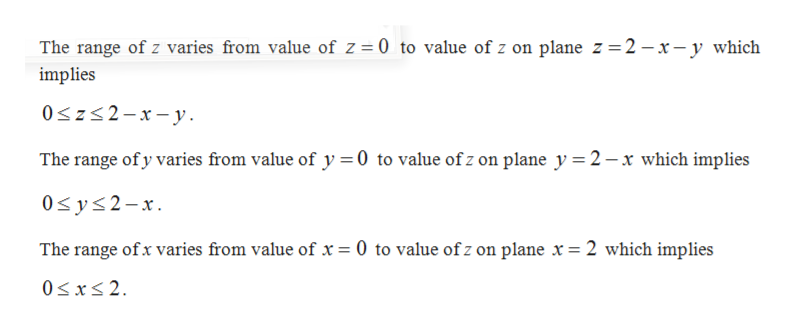# Use the Divergence Theorem to compute the net outward flux of the fieldF=<x,2y,-z> across the surface​ S, where S is the boundary of the tetrahedron in the first octant formed by the plane x+y+z=2

Question
64 views

Use the Divergence Theorem to compute the net outward flux of the field

F=<x,2y,-z> across the surface​ S, where S is the boundary of the tetrahedron in the first octant formed by the plane x+y+z=2

check_circle

Step 1

From the Divergence theorem, for a positively oriented boundary surface (outward Normal) of region E the flux is equal to triple integral of divF over the region E.

Step 2

Find the range of x,y and z as follows. Also observe that the plane is on the first quadrant.help_outlineImage TranscriptioncloseThe range of z varies from value of Z= 0 to value of z on plane z 2 - x-y which implies 0sz2-xy The range of y varies from value of y =0 to value of z on plane y 2-x which implies 0s ys2-x The range ofx varies from value of x = 0 to value ofz on plane x = 2 which implies 0Sxs2 fullscreen
Step 3

The divF can be calculated as fo...

### Want to see the full answer?

See Solution

#### Want to see this answer and more?

Solutions are written by subject experts who are available 24/7. Questions are typically answered within 1 hour.*

See Solution
*Response times may vary by subject and question.
Tagged in

### Integration